# 等边三角形——牵线搭桥我最行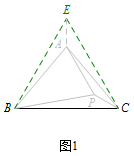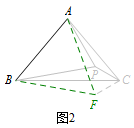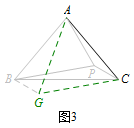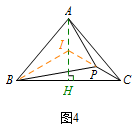《条条大路通罗马》中所给的例题和练习题比较特殊，并不是每题都所有方法皆可，“边边”有缘，总有一款可行．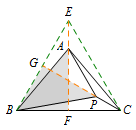延长$EA,CP$，分别与等边$\triangle BCE$的边交于点$F,G$．则$\mathrm{Rt}\triangle ABF\cong \mathrm{Rt}\triangle PGB\ (\mathrm{HL}).$所以$AF=PG,EA=CP.$从而$\triangle BAE \cong \triangle BPC$得证，故$\angle PBC=\angle ABE=10^\circ.$